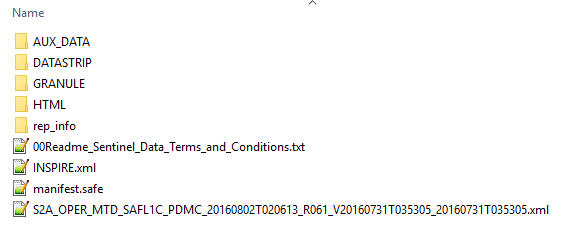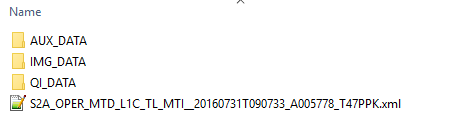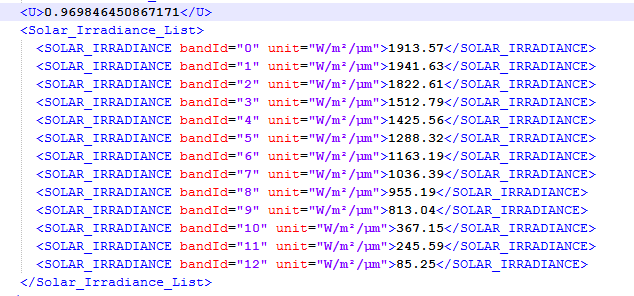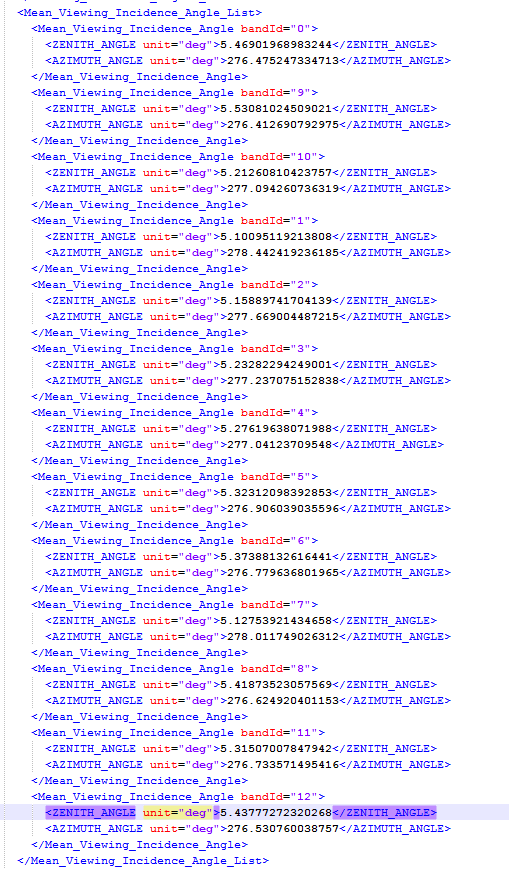# Processing Sentinel 2 Band 12 (SWIR) to Reflectance Values

With TerrSet 18.31 an error in the processing Sentinel 2 data was discovered with the output of Band 12 when calculating reflectance values. The Sentinel 2 import module will only import the raw DN for Band 12 and not calculate reflectance values. This error will be resolved in a future update.

For now, Band 12 will need to be calculated manually using the command line for the module AtmosC. It cannot be run from the AtmosC dialog. We have detailed below the exact parameters to use to construct the command line.

If you are unfamiliar with the IDRISI Macro Language and constructing command line parameters that are used by the module Run Macro, please review the Help system section: IDRISI Macro Language File (.iml) – File Structure.

The parameters needed to process Band 12 to reflectance values are found in the scenes metadata xml files. There are two xml files found under the SAFE folder structure for Sentinel 2. For this example I am using a Sentinel file with the scene ID:

S2A_OPER_PRD_MSIL1C_PDMC_20160802T020613_R061_V20160731T035305_20160731T035305.SAFE

Inside the root of this file, are the file contents:The .xml file in this root folder will be called the “root xml” in the description below.

Inside the Granule folder you will find the second .xml file used to gather parameters needed to process the data. The file used is found root of the granule ID folder, as shown below:The .xml file inside this folder will be called the “granuale xml” in the description below.

To construct the command line, can open these two .xml files in a text editor such as Notepad++.

The command line for AtmosC from the Help states the following parameters:

1: x (to indicate that macro mode is being used)

2: input image (the name of the image file to be corrected)

3: output image (the name of the corrected image)

4: model option (1=apparent reflectance model; 2=dark object subtraction; 3=Cos(t) model; 4=full model)

8: Dn Max (maximum Dn, enter # if radiance option = 2

9: E0 (spectral solar irradiance, see Note 4)

11: sun elevation (0-90; horizon=0)

If model option = 2 or 3:

12: haze Dn (increase in Dn values due to haze)

If model option = 4:

12: haze Dn (increase in Dn values due to haze)

13: optical thickness

14: E downwelling (Spectral diffuse sky irradiance)

We will use the above structure to construct the command line that will look something like this:

Atmosc x S2A_OPER_MSI_L1C_TL_MTI__20160731T090733_A005778_T47PPK_B12* S2A_OPER_MSI_L1C_TL_MTI__20160731T090733_A005778_T47PPK_B12_DOS_Reflectance*2*2*0*.0001*#* 8.2679409936426* 5.43777272320268*66.394365211577*.01

The above macro runs with the example Sentinel file mentioned above. This assumes that the Band 12 file with Raw DN exists in your Working Folder. If not, run the Sentinel 2 import and import the raw DN image for Band 12. The most important parameters to enter are parameters 4 through 12. Pay attention to where these are found in the metadata and how the values are calculated.

The first three parameters are easy enough, with the second parameter the name of the raw DN Band 12. The third parameter will be any output name.

The fourth parameter specifies to use dark object subtraction (DOS) or the Cos(t) model. In my example I specified DOS.

For the fifth parameter, specify 2 to use offset/gain.

For the sixth parameter, specify the offset as: 0

For the seventh parameter, specify the gain as: .0001

For the eighth parameter use the default value of: #

For parameters nine to eleven you will now need to consult the .xml files.

For the ninth parameter, you will need to calculate E0, the solar irradiance. For this parameter for Sentinel 2:

Both solar_irradiance and U values are found in the root xml. Search this .xml file for “solar_irradiance_list”. Use the value for bandId=12 for the solar_irradiance parameter in the equation above. Just above this list is the value of “U”. The ninth parameter is the result of this equation.

The section of the .xml file where these parameters are found are shown here:The tenth parameter is the satellite viewing angle and can be found in the granule xml file. Search this file for the keyword: mean_viewing_incidence_angle_list

Under this section, find bandId=12, and use the Zenith_Angle number. For my example scene, the section in the .xml file looks this:The eleventh parameter is the Sun Elevation and is calculated from the granule xml file. Locate in the file in the Mean_Sun_Angle section. Use the Zenith value and use the equation:

Sun elevation = 90 – zenith angle

For the twelfth parameter, specify .001, as a default minimum valid data value.

Once you construct the macro, you can save it to a text editor, like the module EDIT in TerrSet, save it with a .iml extension, and use Run Macro to output your reflectance band 12. Again, my sample .iml file looks like this:

Atmosc x S2A_OPER_MSI_L1C_TL_MTI__20160731T090733_A005778_T47PPK_B12* S2A_OPER_MSI_L1C_TL_MTI__20160731T090733_A005778_T47PPK_B12_DOS_Reflectance*2*2*0*.0001*#* 8.2679409936426* 5.43777272320268*66.394365211577*.01